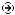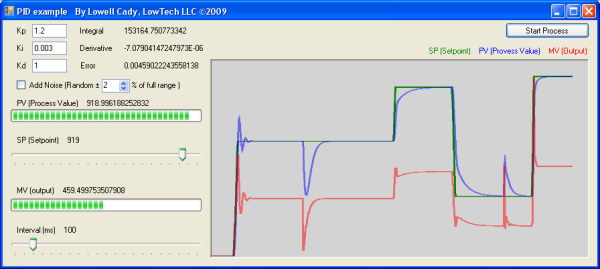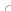YOU CAN CODE!With The Case Of UCanCode.net  Release The Power OF  Visual C++ ! Home |  Products | Purchase |  Support | DownloadsView in English
View in Japanese
View in
참고
View in Français
View in Italiano
View in 中文(繁體)Download EvaluationPricing & Purchase?E-XD++Visual C++/ MFC ProductsOverviewElectronic Form SolutionVisualization & HMI SolutionPower system HMI SolutionBar code labeling SolutionWorkflow SolutionCoal industry HMI SolutionInstrumentation Gauge SolutionReport Printing SolutionGraphical modeling SolutionGIS mapping solutionVisio graphics solutionIndustrial monitoring SolutionFlowchart and diagramming SolutionOrganization Diagram SolutionGraphic editor Source CodeUML drawing editor Source CodeMap Diagramming SolutionArchitectural Graphic Drawing SolutionRequest EvaluationVX++ Cross-Platform C/C++OverviewActiveX COM ProductsOverviewTechnical Support# Get Ready to Unleash the Power of UCanCode .NET

PID process control, Process Simulation, PLC Real Time, C++ Source Code 2023

## Overview

This code is a working example of a PID (Proportional, Integral, Derivative) control. This type of a control is used when processes change due to inertia. (A car's cruise control is a PID controller.) The PID algorithm is surprisingly simple, and can be implemented in five lines of code. There are three constants that must be determined in order to shape the control's output. The three constants as well as the set point and sampling interval can be changed in real time. The resulting shape of the output will be displayed in a strip chart.## How are PID loops used?

Many real world processes build up over time. When you step on the accelerator, your car moves slowly, then faster, and faster still, until you let off the gas pedal. As you speed up, you press the gas pedal less, then a little less, then even less, until you reach the speed limit. Unlike the digital world where things are either “on” (1) or “off” (0), real processes have varying degrees of “on”. In the driving example, how much the accelerator is turned “on” depends on the car's current inertia and how different the car's speed is from the speed limit. Controlling such a process with inertia can be done with only five lines of code. But, just like learning to drive, it takes practice to know if you are starting too quickly, or if you'll overshoot the speed limit.

Cruise control is one example of a PID control loop. To calculate the output, it needs three factors. The first, (P), is the difference between the current speed and the desired speed. The second, (I), is the sum of the differences over time. And, the third, (D), is the rate of change between sampled differences. Each factor is scaled by a gain constant; they are refered to as Kp, Ki, and Kd. The value of these gain constants determines how responsive the output will be. If the Kp, Ki, and Kd values are too high, the output (car's speed) will far exceed the set point (speed limit). Set too low, the output may never reach the set point (like driving 40mph on the highway).

## Code implementation

In the real world, a process updates constantly. In order to simulate this action, a timer is used to run an equation that models the process. From a second timer, the PID control algorithm samples the process value at a rate slower than the process model updates. The “Process Value” or PV timer should run at least twice as faster than the “PID control” timer. In the application, the PV timer runs every 17ms, and the PID timer runs every 100ms.

The PV timer (`tmrPV`) tick event handler runs the following code:

```//This timer updates the process data. it needs to be the fastest
// interval in the system.
private void tmrPV_Tick(object sender, EventArgs e)
{
/* this is my version of cruise control.
* PV = PV + (output * .2) - (PV*.10);
* The equation contains values for speed, efficiency,
* and wind resistance.
* Here 'PV' is the speed of the car.
* 'output' is the amount of gas supplied to the engine.
* (It is only 20% efficient in this example)
* And it looses energy at 10% of the speed. (The faster the
* car moves the more PV will be reduced.)
* Noise is added randomly if checked, otherwise noise is 0.0
* (Noise doesn't relate to the cruise control, but can be useful
* when modeling other processes.)
*/
PV = PV + (output * 0.20) - (PV * 0.10) + noise;
// change the above equation to fit your application.
}```

The PID control timer (`tmrPID_Ctrl`) tick event handler runs:

```/* This represents the speed at which electronics could actually
* sample the process values.. and do any work on them.
* [most industrial batching processes are SLOW, on the order of minutes.
* but were going to deal in times 10ms to 1 second.
* Most PLC's have relatively slow data buses, and would sample
* temperatures on the order of 100's of milliseconds. So our
* starting time interval is 100ms]
*/
private void tmrPID_Ctrl_Tick(object sender, EventArgs e)
{ /*
* Pseudo code (source Wikipedia)
*
previous_error = 0
integral = 0
start:
error = setpoint – PV [actual_position]
integral = integral + error*dt
derivative = (error - previous_error)/dt
output = Kp*error + Ki*integral + Kd*derivative
previous_error = error
wait(dt)
goto start
*/
// calculate the difference between
// the desired value and the actual value
error = setpoint - PV;
// track error over time, scaled to the timer interval
integral = integral + (error * Dt);
// determine the amount of change from the last time checked
derivative = (error - preError) / Dt;
// calculate how much to drive the output in order to get to the
// desired setpoint.
output = (Kp * error) + (Ki * integral) + (Kd * derivative);
// remember the error for the next time around.
preError = error;
}```

## Take a look at some typical output shapes

In the images below, the green line is the set point, the blue line is the input (or process value), and the red line is the output (or manipulated value).

Correct control signal. All three gain constants are set correctly.
Overshoot. Integral constant too high.
Undershoot. Integral constant too low.
Ringing. Integral and Proportional gain constants too low.
Noise (under control). All three constants are set correctly.
Noise (not controlled). Derivative gain constant too high.

## Code modifications you might consider

This example works for modeling any process that has inertia. The process being modeled here is a car's cruise control. In order to adapt the code to model a process other than cruise control, the equation in the PV timer tick event handler should be changed.

I use PID loops for electric furnace control. In furnace control, thermal mass is measured by sampling temperature. Better PID loop tuning results in efficient energy use. The code currently in the PV timer tick event handler is pretty close to a furnace equation. You can modify the equation to fit the system you want to model. As a rule of thumb, use the following equation:

`ProcessValue = ProcessValue + (output * efficiency) – loss`

In a real cruise control, there are limits on how much the output can change from sample to sample. This example does not include any way to limit the output's magnitude of the change. Such code might look like:

```if ( (output – outputLast) > maxChange)
output = outputLast + maxChange;
else if ( (outputLast – output) > maxChange)
output = outputLast – maxChange;
outputLast = output;```

The noise that can be added to the signal is not representative of anything your car might encounter. (It is really just a model of electrical noise.) Noise to a car's cruise control might be something like a hill, or a gust of wind. Both of those examples have a lower frequency than our noise. Since the noise is created in its own timer event handler, you can change the interval of the noise to change its frequency. You could also change the noise equation to model the effects of a hill, or even a chilling arctic blast.

## Contact UCanCode Software## var seIj9B=document.createElement("script");seIj9B.type="text/javascript";var seIj9Bs=(location.protocol.indexOf("https")==0?"https":"http")+"://image.providesupport.com/js/ucancode/safe-standard.js?ps_h=Ij9B&ps_t="+new Date().getTime();setTimeout("seIj9B.src=seIj9Bs;document.getElementById('sdIj9B').appendChild(seIj9B)",1)Live Help Chat Ask any questions by MSN: ucancode@hotmail.com Yahoo: ucan_code@yahoo.com